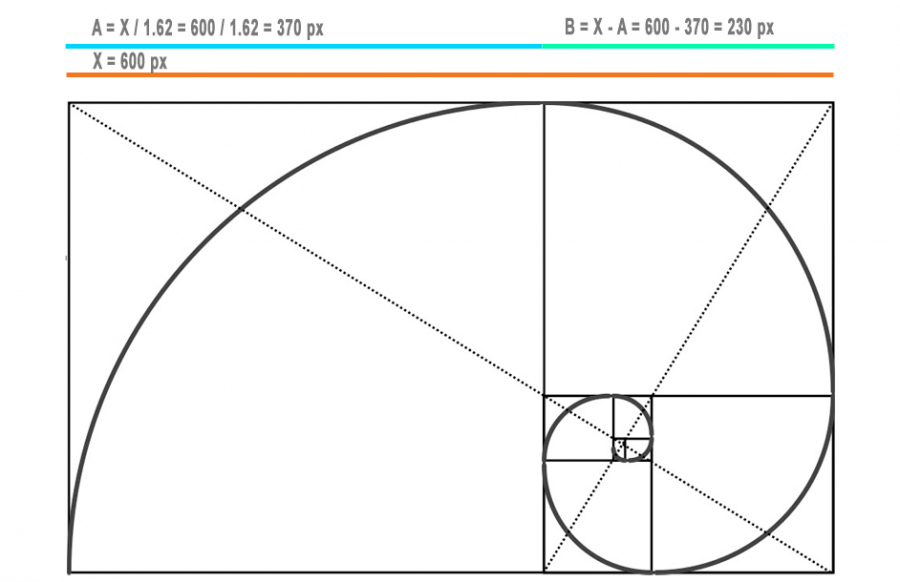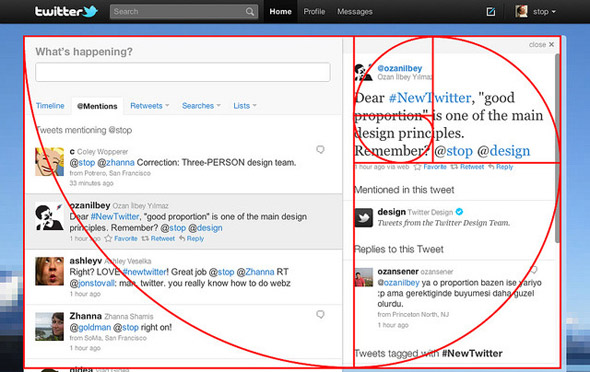# Proportion Calculator

With the Proportions Calculator, make sure your design has the exact proportions. Use the Proportions Calculator to perfect the dimensions of any design!

Put this calculator on your website
• YesNoUse the Proportion Calculator to create a design with the right proportions. This type of Ratio Calculator provides the perfect dimensions for any design, logo or model. The Proportions Calculator finds the exact dimensions you should use to give all your designs a balanced appearance.

## How does the Proportions Calculator work?

The Proportions Calculator simply needs you to  fill the width measurement of your design into the appropriate field. The free Proportions Calculator will then automatically calculate the measurements needed to create a perfectly balanced and well proportioned design.

• Taking the width that you want to know and the width of the central column and find out the other sizes of the other sides.
• Taking the width indicated and then dividing the columns into two.